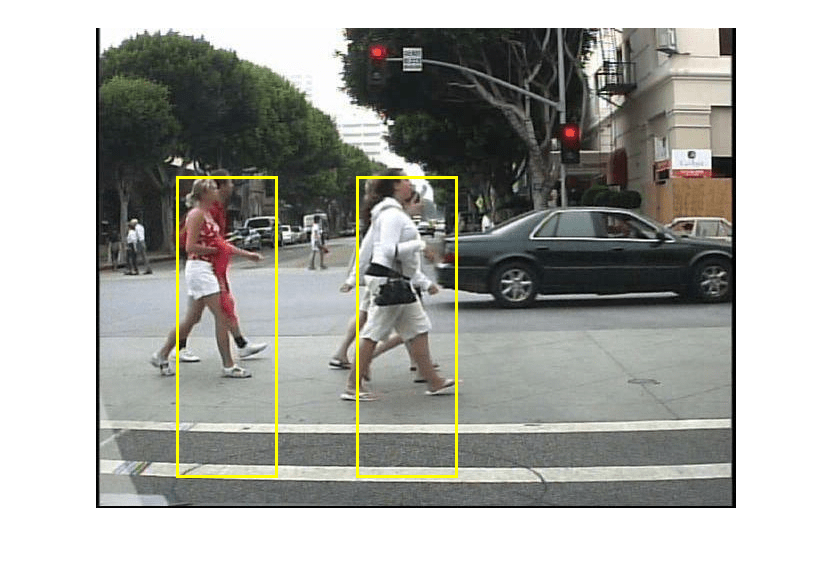Pedestrian Detection

This example shows code generation for pedestrian detection application that uses deep learning. Pedestrian detection is a key issue in computer vision. Pedestrian detection has several applications in the fields of autonomous driving, surveillance, robotics, and so on.

Third-Party Prerequisites

Required

This example generates CUDA® MEX and has the following third-party requirements.

• CUDA enabled NVIDIA® GPU and compatible driver.

Optional

For non-MEX builds such as static, dynamic libraries or executables, this example has the following additional requirements.

Verify GPU Environment

Use the coder.checkGpuInstall function to verify that the compilers and libraries necessary for running this example are set up correctly.

envCfg = coder.gpuEnvConfig('host');
envCfg.DeepLibTarget = 'cudnn';
envCfg.DeepCodegen = 1;
envCfg.Quiet = 1;
coder.checkGpuInstall(envCfg);

The Pedestrian Detection Network

The pedestrian detection network was trained by using images of pedestrians and non-pedestrians. This network is trained in MATLAB® by using the trainPedNet.m helper script. A sliding window approach crops patches from an image of size [64 32]. Patch dimensions are obtained from a heatmap, which represents the distribution of pedestrians in the images in the data set. It indicates the presence of pedestrians at various scales and locations in the images. In this example, patches of pedestrians close to the camera are cropped and processed. Non-Maximal Suppression (NMS) is applied on the obtained patches to merge them and detect complete pedestrians.The pedestrian detection network contains 12 layers which include convolution, fully connected, and classification output layers.

Use the analyzeNetwork (Deep Learning Toolbox) function to display an interactive visualization of the deep learning network architecture.

analyzeNetwork(PedNet);

The pedDetect_predict Entry-Point Function

The pedDetect_predict.m entry-point function takes an image input and performs prediction on an image by using the deep learning network saved in the PedNet.mat file. The function loads the network object from the PedNet.mat file into a persistent variable pednet. Then function reuses the persistent object on subsequent calls.

type('pedDetect_predict.m')
function selectedBbox = pedDetect_predict(img)
%#codegen

% Copyright 2017-2021 The MathWorks, Inc.

coder.gpu.kernelfun;

persistent pednet;
if isempty(pednet)
end

[imgHt , imgWd , ~] = size(img);
VrHt = [imgHt - 30 , imgHt]; % Two bands of vertical heights are considered

% patchHt and patchWd are obtained from heat maps (heat map here refers to
% pedestrians data represented in the form of a map with different
% colors. Different colors indicate presence of pedestrians at various
% scales).
patchHt = 300;
patchWd = patchHt/3;

% PatchCount is used to estimate number of patches per image
PatchCount = ((imgWd - patchWd)/20) + 2;
maxPatchCount = PatchCount * 2;
Itmp = zeros(64 , 32 , 3 , maxPatchCount);
ltMin = zeros(maxPatchCount);
lttop = zeros(maxPatchCount);

idx = 1; % To count number of image patches obtained from sliding window
cnt = 1; % To count number of patches predicted as pedestrians

bbox = zeros(maxPatchCount , 4);
value = zeros(maxPatchCount , 1);

%% Region proposal for two bands
for VrStride = 1 : 2
% Obtain horizontal patches with stride 20.
for HrStride = 1 : 20 : (imgWd - 60)
ltMin(idx) = HrStride + 1;
rtMax = min(ltMin(idx) + patchWd , imgWd);
lttop(idx) = (VrHt(VrStride) - patchHt);
It = img(lttop(idx): VrHt(VrStride) , ltMin(idx) : rtMax , :);
Itmp(:,:,:,idx) = imresize(It,[64,32]);
idx = idx + 1;
end
end

for j = 1 : size (Itmp,4)
score = pednet.predict(Itmp(:,:,:,j)); % Classify ROI
% accuracy of detected box should be greater than 0.90
if (score(1,2) > 0.80)
bbox(cnt,:) = [ltMin(j),lttop(j), patchWd , patchHt];
value(cnt,:) = score(1,2);
cnt = cnt + 1;
end

end

%% NMS to merge similar boxes
if ~isempty(bbox)
[selectedBbox,~] = selectStrongestBbox(bbox(1:cnt-1,:),...
value(1:cnt-1,:),'OverlapThreshold',0.002);
end

Generate CUDA MEX for the pedDetect_predict Function

Create a GPU Configuration object for a MEX target and set the target language to C++. Use the coder.DeepLearningConfig function to create a CuDNN deep learning configuration object and assign it to the DeepLearningConfig property of the GPU code configuration object. To generate CUDA MEX, use the codegen command and specify the size of the input image. This value corresponds to the input layer size of pedestrian detection network.

im = imresize(im,[480,640]);

cfg = coder.gpuConfig('mex');
cfg.TargetLang = 'C++';
cfg.DeepLearningConfig = coder.DeepLearningConfig('cudnn');
codegen -config cfg pedDetect_predict -args {im} -report
Code generation successful: View report

Run Generated MEX

Call pednet_predict_mex on the input image.

imshow(im);ped_bboxes = pedDetect_predict_mex(im);

Display the final predictions.

outputImage = insertShape(im,'Rectangle',ped_bboxes,'LineWidth',3);
imshow(outputImage);Classification on Video

The included helper file pedDetect_predict.m grabs frames from a video, performs prediction, and displays the classification results on each of the captured video frames.

fps = 0;
while hasFrame(v)
im = imresize(im,[480,640]);
% Call MEX function for pednet prediction
tic;
ped_bboxes = pedDetect_predict_mex(im);
newt = toc;
% fps
fps = .9*fps + .1*(1/newt);
% display
outputImage = insertShape(im,'Rectangle',ped_bboxes,'LineWidth',3);
imshow(outputImage)
pause(0.2)
end

Clear the static network object that was loaded in memory.

clear mex;